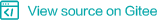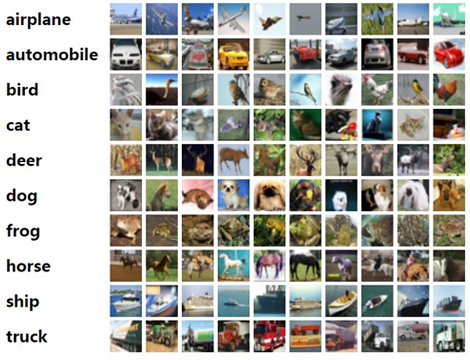# 计算机视觉应用¶## 图像分类¶

```def classify(image):
label = model(image)
return label
```

MindSpore预置了典型的卷积神经网络，开发者可以直接使用，如LeNet，使用方式如下：

```from mindspore.model_zoo.lenet import LeNet5
network = LeNet(num_classes)
```

MindSpore当前支持的图像分类网络包括：典型网络LeNet、AlexNet、ResNet。

## 任务描述及准备¶1. 下载CIFAR-10数据集

2. 数据加载和预处理

3. 定义卷积神经网络，本例采用ResNet-50网络

4. 定义损失函数和优化器

5. 调用`Model`高阶API进行训练和保存模型文件

6. 加载保存的模型进行推理

## 下载CIFAR-10数据集¶

```wget https://www.cs.toronto.edu/~kriz/cifar-10-binary.tar.gz
```

```tar -zvxf cifar-10-binary.tar.gz
```

### 数据预加载和预处理¶

1. 加载数据集

数据加载可以通过内置数据集格式`Cifar10Dataset`接口完成。

`Cifar10Dataset`，读取类型为随机读取，内置CIFAR-10数据集，包含图像和标签，图像格式默认为uint8，标签数据格式默认为uint32。更多说明请查看API中`Cifar10Dataset`接口说明。

数据加载代码如下，其中`data_home`为数据存储位置：

```cifar_ds = ds.Cifar10Dataset(data_home)
```
2. 数据增强

数据增强主要是对数据进行归一化和丰富数据样本数量。常见的数据增强方式包括裁剪、翻转、色彩变化等等。MindSpore通过调用`map`方法在图片上执行增强操作：

```resize_height = 224
resize_width = 224
rescale = 1.0 / 255.0
shift = 0.0

# define map operations
random_crop_op = C.RandomCrop((32, 32), (4, 4, 4, 4)) # padding_mode default CONSTANT
random_horizontal_op = C.RandomHorizontalFlip()
resize_op = C.Resize((resize_height, resize_width)) # interpolation default BILINEAR
rescale_op = C.Rescale(rescale, shift)
normalize_op = C.Normalize((0.4914, 0.4822, 0.4465), (0.2023, 0.1994, 0.2010))
changeswap_op = C.HWC2CHW()
type_cast_op = C2.TypeCast(mstype.int32)

c_trans = []
if training:
c_trans = [random_crop_op, random_horizontal_op]
c_trans += [resize_op, rescale_op, normalize_op, changeswap_op]

# apply map operations on images
cifar_ds = cifar_ds.map(input_columns="label", operations=type_cast_op)
cifar_ds = cifar_ds.map(input_columns="image", operations=c_trans)
```
3. 数据混洗和批处理

最后通过数据混洗（shuffle）随机打乱数据的顺序，并按batch读取数据，进行模型训练：

```# apply shuffle operations
cifar_ds = cifar_ds.shuffle(buffer_size=10)

# apply batch operations
cifar_ds = cifar_ds.batch(batch_size=args_opt.batch_size, drop_remainder=True)

# apply repeat operations
cifar_ds = cifar_ds.repeat(repeat_num)
```

### 定义卷积神经网络¶

ResNet通常是较好的选择。首先，它足够深，常见的有34层，50层，101层。通常层次越深，表征能力越强，分类准确率越高。其次，可学习，采用了残差结构，通过shortcut连接把低层直接跟高层相连，解决了反向传播过程中因为网络太深造成的梯度消失问题。此外，ResNet网络的性能很好，既表现为识别的准确率，也包括它本身模型的大小和参数量。

MindSpore Model Zoo中已经内置了ResNet模型，可以采用ResNet-50网络，调用方法如下：

```from mindspore.model_zoo.resnet import resnet50
network = resnet50(class_num=10)
```

### 定义损失函数和优化器¶

MindSpore中定义损失函数和优化器的代码样例如下：

```# loss function definition

# optimization definition
opt = Momentum(filter(lambda x: x.requires_grad, net.get_parameters()), 0.01, 0.9)
```

### 调用`Model`高阶API进行训练和保存模型文件¶

```'''
network, loss, optimizer are defined before.
batch_num, epoch_size are training parameters.
'''
model = Model(net, loss_fn=ls, optimizer=opt, metrics={'acc'})

# CheckPoint CallBack definition
config_ck = CheckpointConfig(save_checkpoint_steps=batch_num, keep_checkpoint_max=35)
ckpoint_cb = ModelCheckpoint(prefix="train_resnet_cifar10", directory="./", config=config_ck)

# LossMonitor is used to print loss value on screen
loss_cb = LossMonitor()
model.train(epoch_size, dataset, callbacks=[ckpoint_cb, loss_cb])
```

### 加载保存的模型，并进行验证¶

```param_dict = load_checkpoint(args_opt.checkpoint_path)